# LearnDash Multi Quiz Import Export - Overview

## Installation / Setup Instruction:

The following are the step by step instructions to setup LearnDash multiple quiz import/export addon.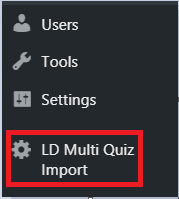# How To Export Quiz:

When the Learndash Quiz Import/Export addon is activated then options of  Export as XLS and Export as XLSX become available in the Quizzes columns. To export the quiz we can simply click on the export button and it will download the quiz. With the help of the add-on, we can import it to another site.

We can also bulk export quizzes in  .xls or in .xlxs format.

How to Export Quiz Tutorial: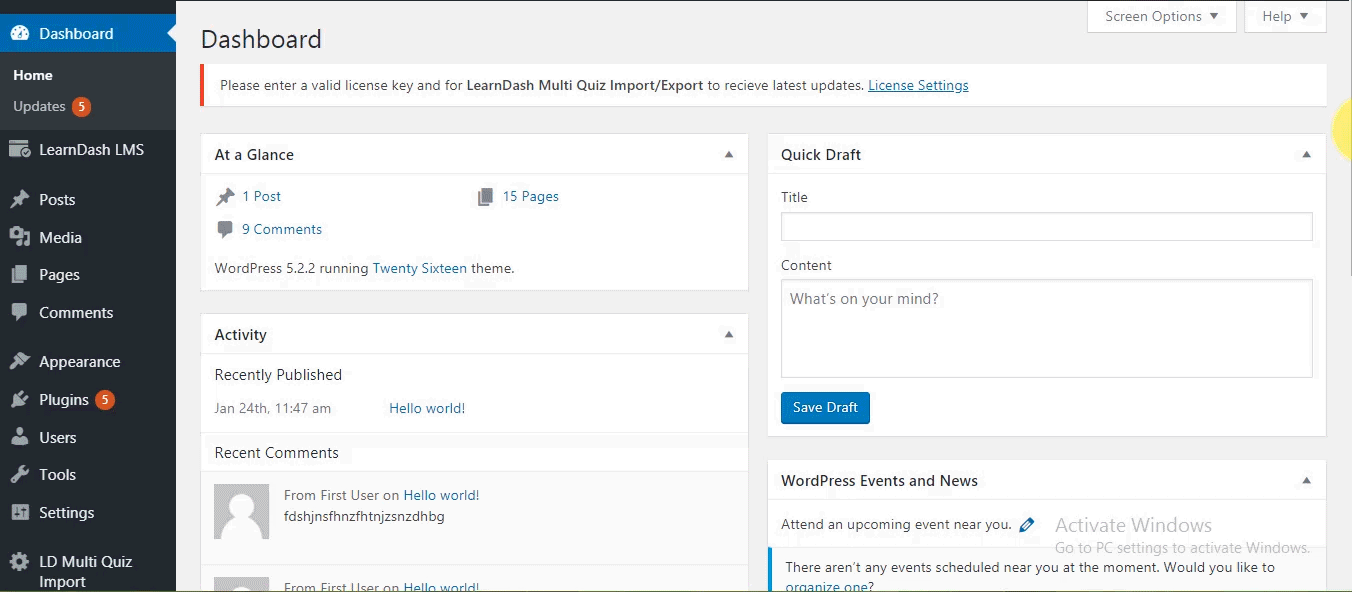# LD Quiz Import:

This tab shows the input area for the quiz file to be imported. Select the Microsoft Excel. xls or .xlxs file and click on the import quiz button. Multiple quizzes can be imported at once.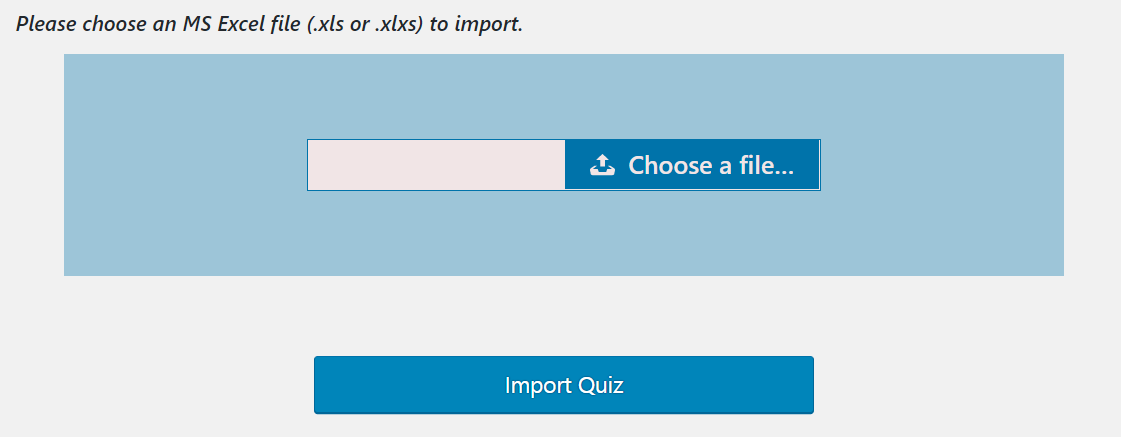Note: Multiple quizzes (sheets) can be imported in a single import. Scroll down to see the video.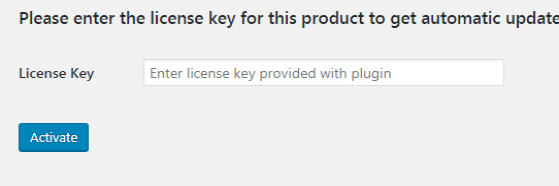# Import/Export Settings:

All the default LearnDash quiz options are present here in different tabs. So the new imported quiz will adopt the quiz options settings from here.

### General Option

Description:

Here the admin can add the quiz description for the imported quiz.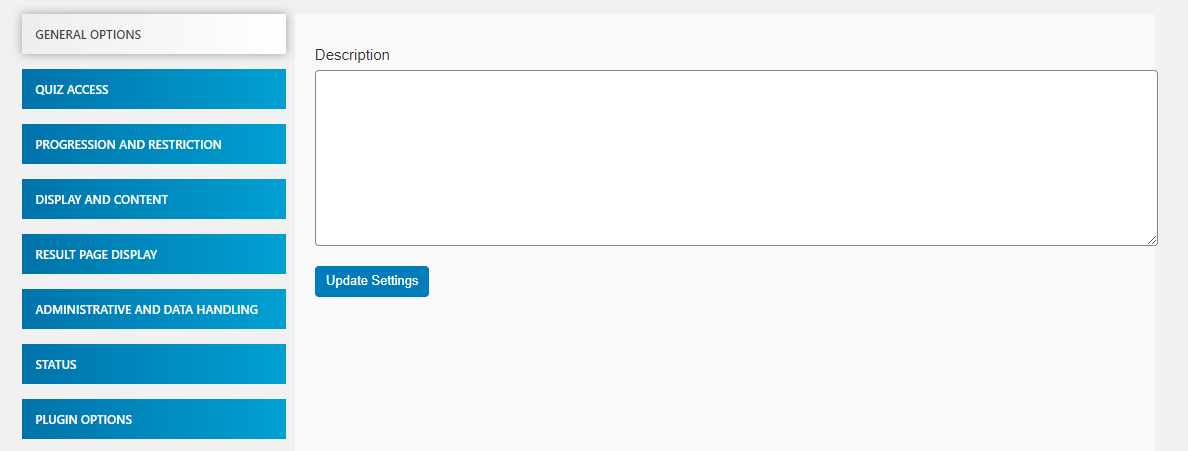### Quiz Access

Quiz Prerequisites:

The admin can use this option to set a prerequisites quiz/quizzes for a newly imported quiz.

Allowed Users:

This option is especially useful if administering quizzes via shortcodes on non-course pages, or if your course is open but you wish only authenticated users to take the quiz.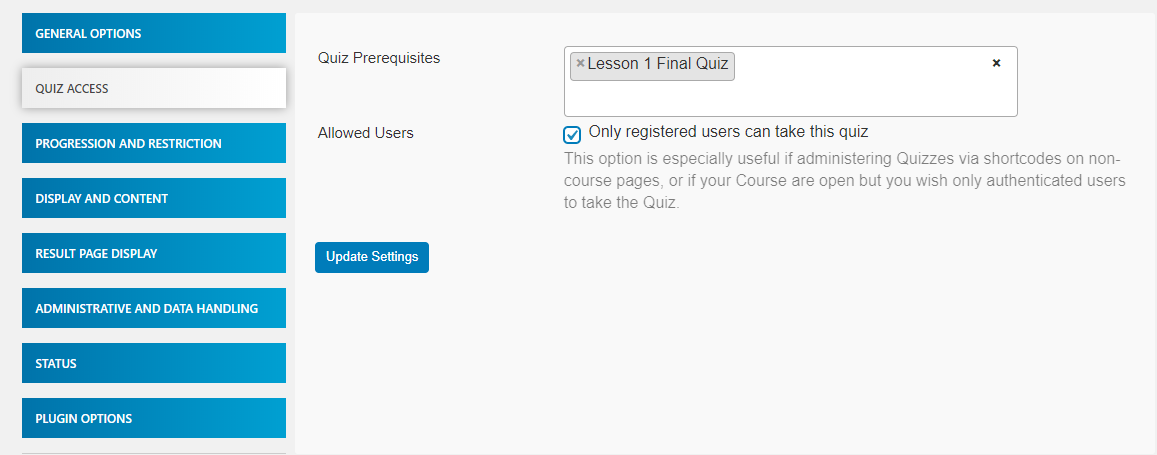### Progression and Restriction

Restrict Quiz Retakes:

By enabling this option the admin can restrict the quiz retake.

• The Number of Retakes Allowed: This option allows the admin to select the number of retries allowed. If the admin selects 1, the user can take this quiz 2 times.
• Retries Applicable to: This option allows the admin to select the users (i.e  All users or Registered users only or Anonymous user only) to allow them to retake the quiz.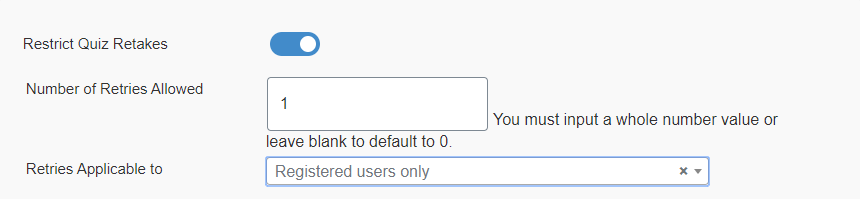Question Completion:

If this option is  checked, then the user must have to complete all the questions of the quiz.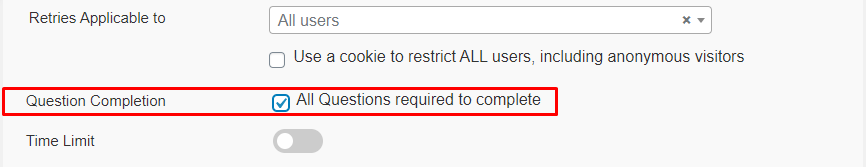Time Limit:

This option allows the admin to add a time limit (HH-MM-SS) for the quiz. The quiz will automatically be submitted after the select time period.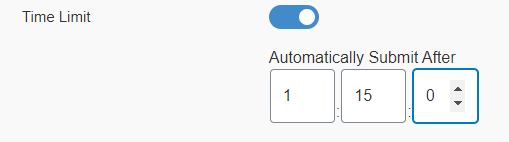### Display and Content

Auto Start:

If you enable this option, the quiz will start automatically after the page is loaded.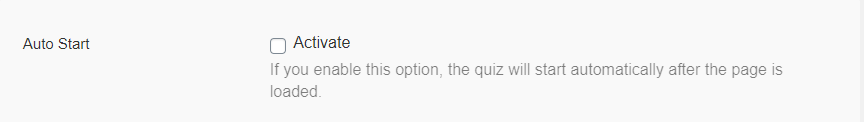Question Display:

The admin can set the question Display setting here. This drop-down has two options:  One question at a time and All questions at once.

You can further set these options as per your requirement.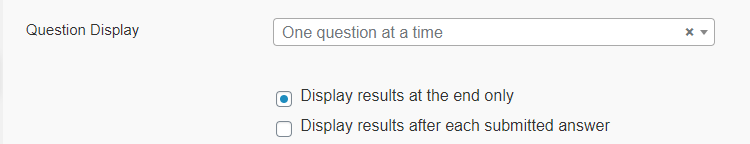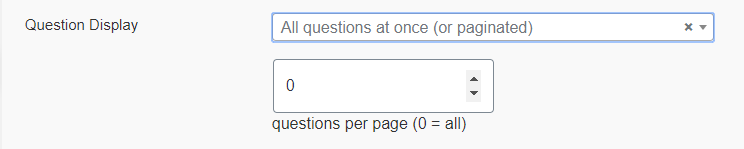Question Overview Table:

This option allows the admin to show a Question Overview Table on the quiz page.

If the user has answered the question, The question cell from the table will turn blue. The user can also jump to the specific question by clicking on the question cell from the table.

Backend: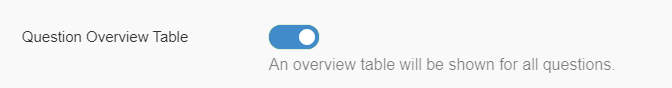Frontend: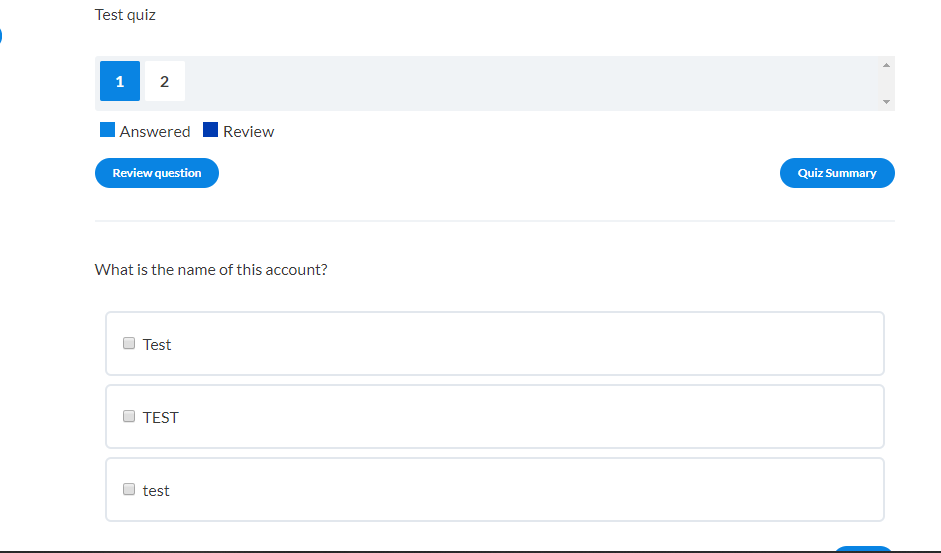• Quiz Summary:

If this option is enabled, a  Quiz Summary button will be visible on the quiz page. On clicking this button the user can view his/her quiz summary.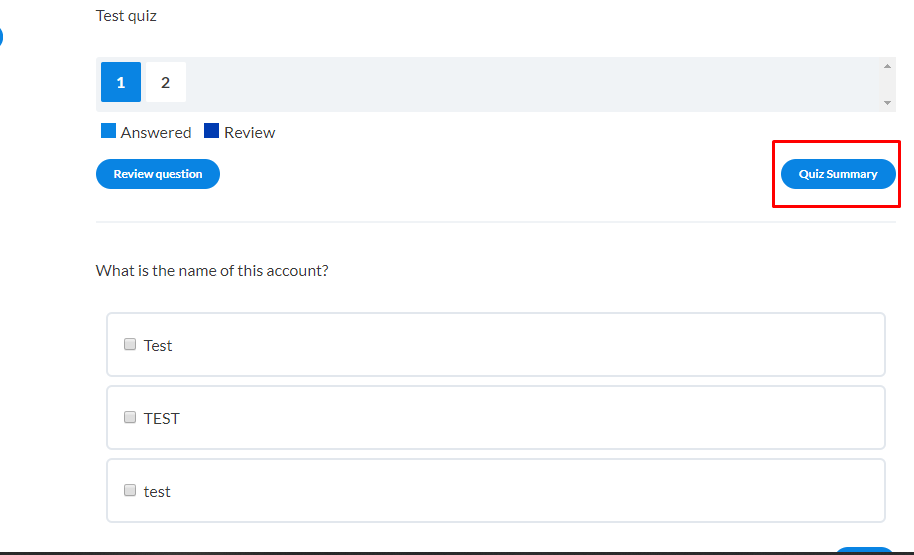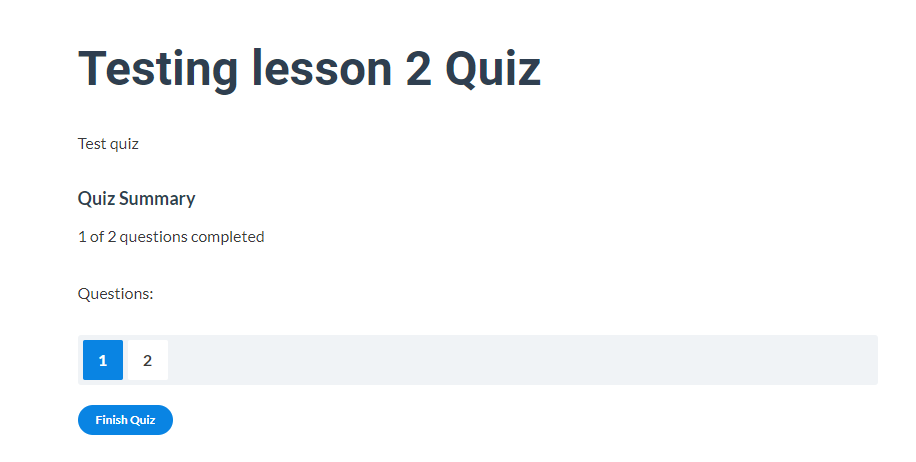• Skip Question:

If this option is enabled, the user can skip any question of the quiz.

Custom Question Ordering:

This option allows the admin to customize the order of the quiz questions.

• Sort by Category:

If this option is checked, the questions will be ordered by their category type.

• Randomize Order:

If this option is enabled, the admin can randomize the order of the questions.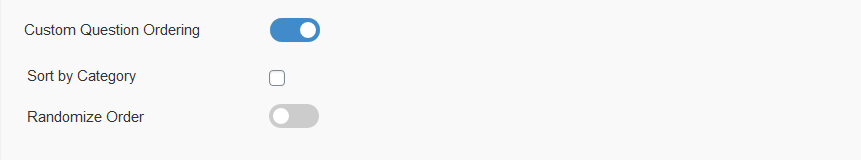If this option is enabled, the admin can show (Point Value, Question Category, Question Position, Question Numbering, Number Answers) all or any of these at the user end at the quiz page.

The admin can also randomize the answers by enabling the  Randomize Answers option under the Additional Question Options.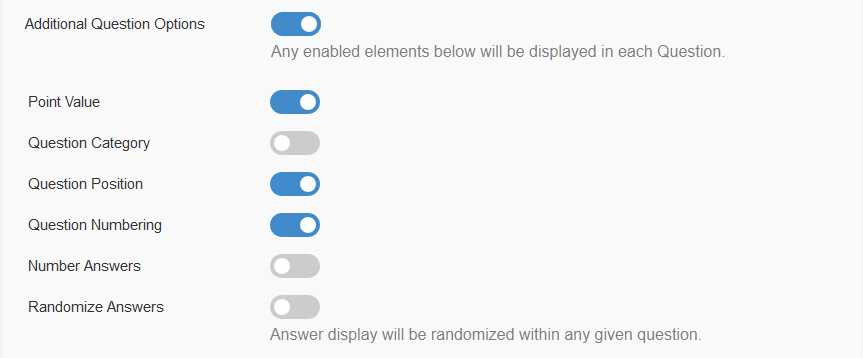Quiz Title:

By enabling this option, the second quiz title will be displayed on the quiz post. This option is recommended if displaying quizzes via Shortcode.

### Result Page Display

Result Message(s):

When enabled, the first message will be displayed to all users once the quiz ends.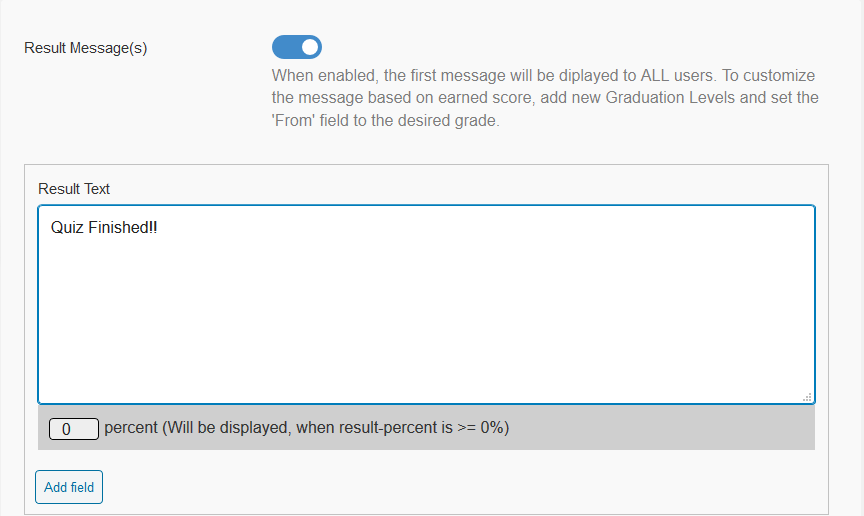To customize the message based on the earned score, Click at  Add field button, add new graduation levels’ and set the 'from' field to the desired grade.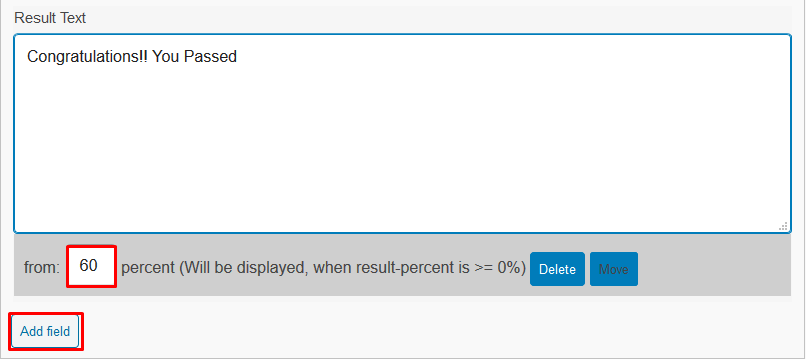Restart Quiz button:

If this option is enabled, a quiz restart button will be shown on the quiz page. On clicking the user can restart the quiz.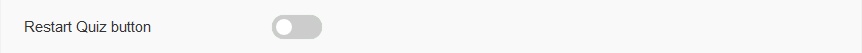Custom Results Display:

Enable the items (such as Average Score, Category Score, Overall Score, No. of Correct Answers, and Time Spent) you wish to display on the Result Page.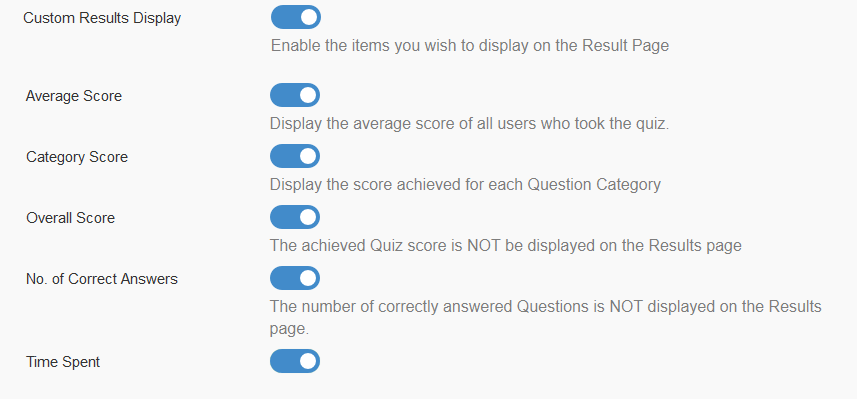If this option is enabled, the admin can select which data (for example Correct / Incorrect Messages, Correct / Incorrect Answer Marks, View Questions Button) users should be able to view when reviewing their submitted questions.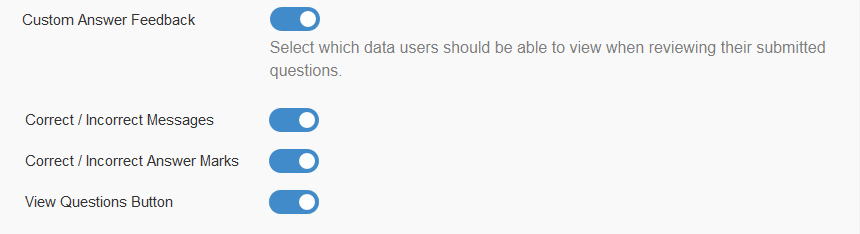Custom Fields:

Enable this option to gather data from your users before or after the quiz. All data is stored in the Quiz Statistics.

You can add multiple fields. You can also select the display position (i.e On the quiz start page or At the end of the quiz) of that form.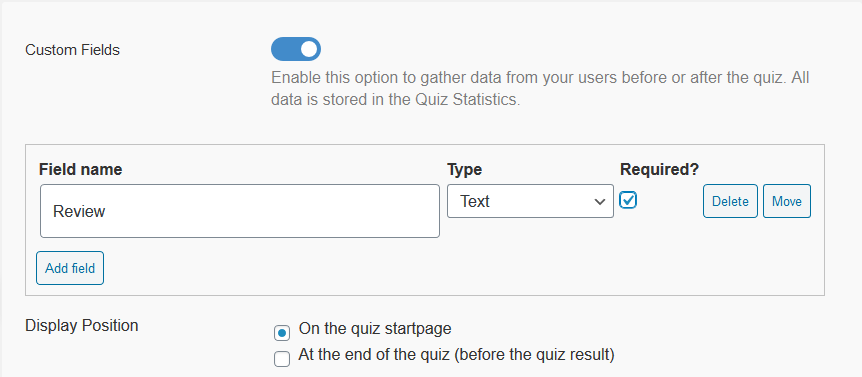Enable this option if you want to display the Leaderboard. You can also configure further options for the leaderboard here.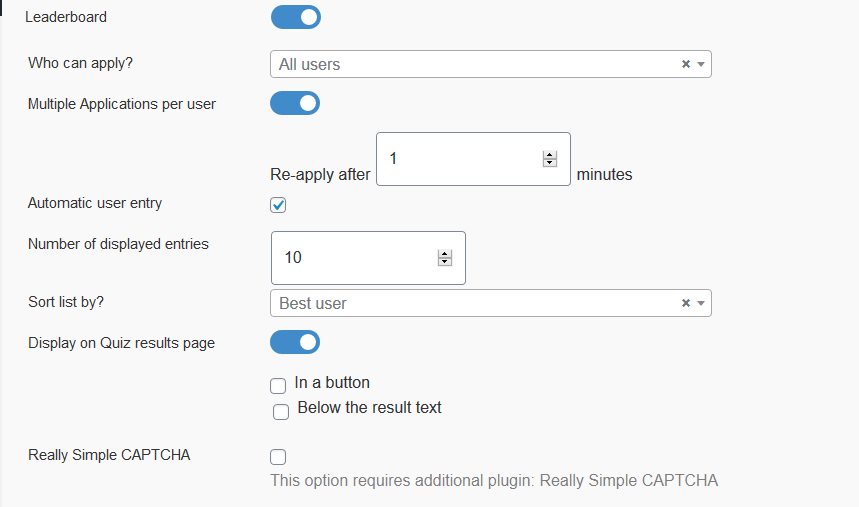Quiz Statistics:

Enable this option if you want to display the quiz statistics.

• Front-end Profile Display:
• Statistics IP-lock:

Protect the statistics from spam. Results will only be saved every X minutes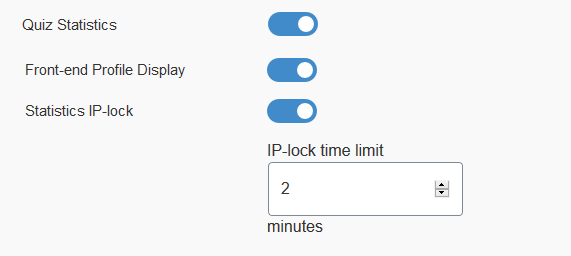Enable this option if you want to enable email notifications. You can enable email notifications for admin and users both.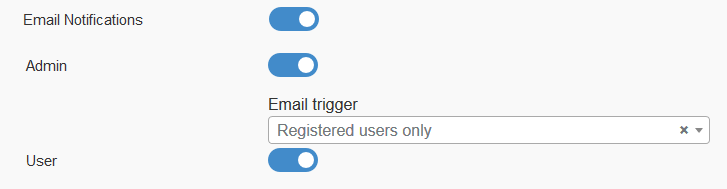• Associated Settings: Via this option, you can associate these settings with any LearnDash quiz.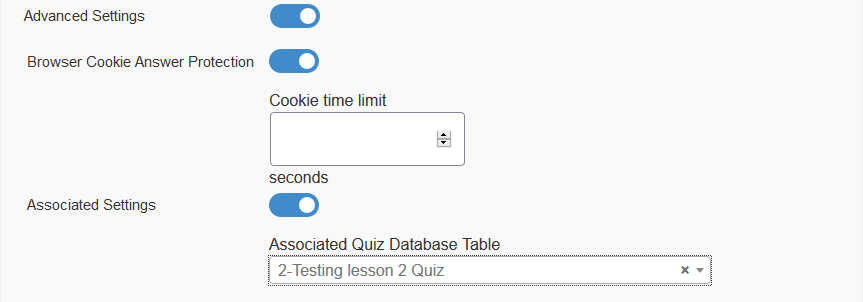## Status:

This tab shows the count of imported and exported quizzes.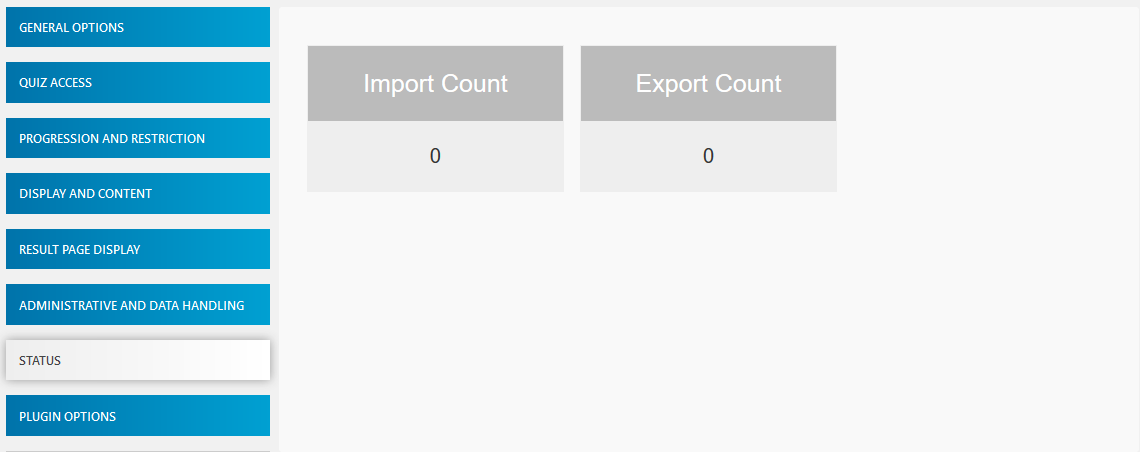## Plugin Options:

### Minimum Role To Administer Plugin

This dropdown lets the selection of the role who can access and use this plugin. If we want any teacher or Instructor to prepare and import quizzes we can select the role respectively.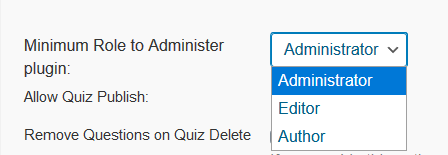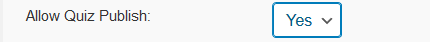### Remove Questions on Quiz Delete

This option lets the questions to be discarded when the associated quiz is deleted by the admin automatically.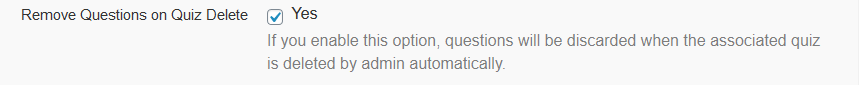### Discard Old Questions in Import

This tab shows the option to discard previously imported questions of the same quiz.

If we import a quiz, let’s call it  Final Quiz, with questions A, B, C and D, and if we want to import more questions  E, F and G of this quiz with the Discard Old Questions in Import option as checked, after importing the questions, the old questions i.e A, B, C, and D will be discarded and only new questions i.e E, F and G will remain.

If the  Discard Old Questions in Import option is unchecked, then all questions will be there in the quiz i.e A, B, C, D, E, F, and G.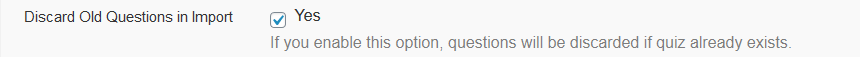### Allow Import of Existing Questions

Enabling this option will let you have an extra step to show up during the import process to choose existing questions for import.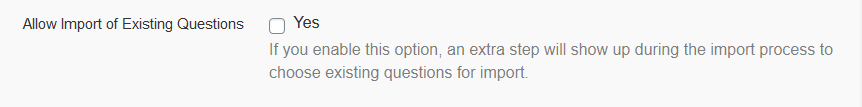How to Import Quiz: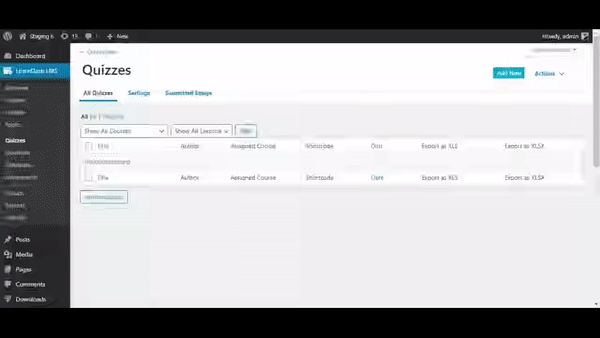# Latex Text:

Added the latex text support for math equations in LearnDash quiz questions.

There are three steps to use latex in your LearnDash quiz.

1. Install the free MathJax LaTeX plugin
3. Create a quiz with latex text

This format should be needed to use the latex text $E=mc^2$

Note: Follow this article Using LaTeX in LearnDash to use the latex text in LearnDash quiz questions.

# LearnDash Multiple Quiz Import/Export Template Guide

LearnDash Multiple Quiz Import/Export uses two standard templates to import and export all LearnDash question types. These templates should be followed in order to create your own templates for import/export.

The two Standard Templates (one for .xls and other for .xlxs) can be found in the plugin folder:

Learndash-multi-quiz-import-export > Documentation > Standard Template

The template contains the standard LearnDash question field names as columns.

It contains the following columns which would translate to a LearnDash quiz questions:

## 1. Quiz Title

This column represents the quiz title. If this column is empty then the quiz will adopt the title from the sheet name.

## 2. Question Type

This column represents the type of LearnDash question. This column can

have the following values corresponding to the LearnDash question types.

Single  => Single choice

Multiple => Multiple choice

cloze_answer  => Fill in the blank

essay => Essay / Open Answer

## 3. Category

The column should contain the value for the LearnDash question category field.

## 4. Title

This represents the question title for a LearnDash quiz question.

## 5. Total Points

The column represents the total points for each answer. It can be left empty for all question types except the  essay type questions. For all other question types, the total points are calculated from their individual answers. This column is only significant for essay type questions since it is used to assign points to each essay question.

## 6. Different Points for each answer

It should be set to  yes if you need to set different points for each answer and no If you want to set a standard point for all answers.

## 7. Question Text

This column would fill up the question text area field for the question.

In LearnDash, there is an option to add media to the answers for some question types. If you want to display your answer with image and text, then set the value as  HTML for this column, otherwise else set the value as text.

Note: If we select HTML on matrix sorting question, then it will apply on both fields i.e element and criterion.

## 9. Answer # and Point # Columns

These columns should be set according to the maximum number of answers present in any of the questions for the particular quiz import.

If a quiz contains 13 answers for a particular question inside it, there  should be 13 columns in the template.

From:

Point 1 – Point 13

This column will contain all the answers which are available for a question. If a question has less number of answers than the maximum answer # column, then simply leave that column as empty. The point # column associates points with each answer (If  different points for each answer is enabled).

Note:

1. For a matrix sort answer the value inside these answer columns should be in

this format  {criterion}{element}

2. To put an image as an answer the value inside these answer columns should be

in this format  [image_url]

After the individual answer and points column, there is again a column named  Answer. This column serves multiple purposes.

single choice

→ For a single choice answer, it represents the correct answer from all the choices.

multiple choice

→ For a multiple-choice answer, It represents the correct answer(s) from all the choices. The pattern to indicate the correct choice is as follows:

The pipe (|) sign is used to indicate the answer number and if the answer is not correct there is no value between the pipes. For the correct answer, answer # should be entered.

For.eg :

||2||4||6 → This indicates that answer # 2, 4, and 6 are correct for this multiple choice question and answers # 1, 3, and 5 are incorrect.

Free choice

→ For a free choice answer, it represents the single answer for this question type, as there can only be one answer. The answers can, however, be separated through line break. To add multiple answers for a free choice using this column, you need to add line breaks in the cell for this column.

For.eg:

value1

value2

value3

(To add a line break in excel file use ALT + Enter to selected column cell)

Assessment

→ For an assessment answer, It represents the values for the single answer for this question type. The answer should be in the following format:

{[ans1][ans2][ans3]}

Fill In the Blank

→ For a close (fill in the blanks) answer, it represents value for the single answer for this question type. The answer should be in the following format:

This is a sample {cloze|5} question and It represents a sample cloze {type} question.

Note: A blank answer should be enclosed in {} and to enable separate points for each blank answer, the point has to be added after the pipe (|). If there is no separate point for each blank then there is no need to add the pipe (|) symbol.

Essay

→ For an essay answer, it represents the answer submit method and the grading criteria. The following combination of values can be added to set the above values for this answer type.

Text submit, not graded and full points

Text submit, graded and full points

Text submit, not graded and no points

This corresponds to the total number of answer columns in the sheet. Set this column value according to the maximum number of answers present in the sheet.

## 12. Message with correct answer

This text will be visible if a question is answered correctly. It can be used as an explanation for complex questions.

## 13. Message with incorrect answer

This text will be visible if a question is answered incorrectly. It can be used as an explanation for complex questions.

## 14. Hint

Here you can enter the solution hint, for the answer.

## 15. Materials

Here you can enter text material, for the quiz.

## 16. Repeats

Here you can enter how many numbers of times a user can take this quiz e.g if you enter 1, the user can take this quiz 2 times.

## 17. Certificate Threshold

Here you can enter how much percentage is compulsory to earn the associated certificate.

## 18. Passing Percentage

Here you can enter the quiz passing percentage.

## 19. Course

In this column, you have to enter the course id of the course to whom you want to associate that quiz.

## 20. Lesson or Topic

In this column, you have to enter the id of the lesson or topic to whom you want to associate that quiz.

## 21. Certificate

Here you can enter the certificate id of the certificate you want to associate with that quiz.